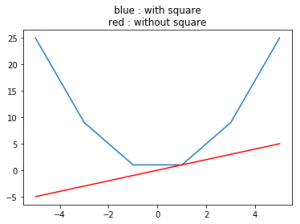# numpy.square() in Python

## numpy.square() in Python

numpy.square(arr, out = None, ufunc ‘square’) : This mathematical function helps user to calculate square value of each element in the array.

Parameters :

```arr  : [array_like] Input array or object
whose elements, we need to square.
```

Return :

```An array with square value of each array.
```

Code #1 : Working

 `# Python program explaining` `# square () function` ` ` `import` `numpy as np` ` ` `arr1 ``=` `[``1``, ``-``3``, ``15``, ``-``466``]` `print` `(``"Square Value of arr1 : \n"``, np.square(arr1))` ` ` `arr2 ``=` `[``23` `,``-``56``]` `print` `(``"\nSquare Value of arr2 : "``, np.square(arr2))`

Output :

```Square Value of arr1 :
[     1      9    225 217156]

Square Value of arr2 :  [ 529 3136]
```

Code #2 : Working with complex numbers

 `# Python program explaining` `# square () function` ` ` `import` `numpy as np` ` ` `a ``=` `4` `+` `3j` `print``(``"Square(4 + 3j) : "``, np.square(a))` ` ` `b ``=` `16` `+` `13j` `print``(``"\nSquare value(16 + 13j) : "``, np.square(b))`

Output :

```Square(4 + 3j) :  (7+24j)

Square value(16 + 13j) :  (87+416j)```

Code #3 : Graphical Representation of numpy.square()

 `# Python program explaining` `# square () function` ` ` `import` `numpy as np` `import` `matplotlib.pyplot as plt` ` ` `a ``=` `np.linspace(start ``=` `-``5``, stop ``=` `5``,` `                ``num ``=` `6``, endpoint ``=` `True``)` `                 ` `print``(``"Graphical Representation : \n"``, np.square(a))` ` ` `plt.title(``"blue : with square\nred : without square"``)` `plt.plot(a, np.square(a))` ` ` `plt.plot(a, a, color ``=` `'red'``)` `plt.show()`

Output :

```Graphical Representation :
[ 25.   9.   1.   1.   9.  25.]
```References :
https://docs.scipy.org/doc/numpy-1.13.0/reference/generated/numpy.absolute.html

Last Updated on March 1, 2022 by admin

## Get all rows in a Pandas DataFrame containing given substringGet all rows in a Pandas DataFrame containing given substring

Get all rows in a Pandas DataFrame containing given substring Let’s see how to get

## Generating all possible Subsequences using RecursionGenerating all possible Subsequences using Recursion

Generating all possible Subsequences using Recursion Given an array. The task is to generate and

## Q-Learning in PythonQ-Learning in Python

Q-Learning in Python Reinforcement Learning briefly is a paradigm of Learning Process in which a learning

## How to Plot Mean and Standard Deviation in Pandas?How to Plot Mean and Standard Deviation in Pandas?

How to Plot Mean and Standard Deviation in Pandas? Errorbar is the plotted chart that refers

## Python – Display images with PyGamePython – Display images with PyGame

Python | Display images with PyGame Pygame is a cross-platform set of Python modules designed for

## Saving a Video using OpenCVSaving a Video using OpenCV

Saving a Video using OpenCV OpenCV is an open-source and most popular computer vision library

## Python – Itertools.chain()Python – Itertools.chain()

Python – Itertools.chain() The itertools is a module in Python having a collection of functions that are

## How to read multiple text files from folder in Python?How to read multiple text files from folder in Python?

How to read multiple text files from folder in Python? Python is a strong language